9 Financial Mathematics

# 9.3 Interest Rates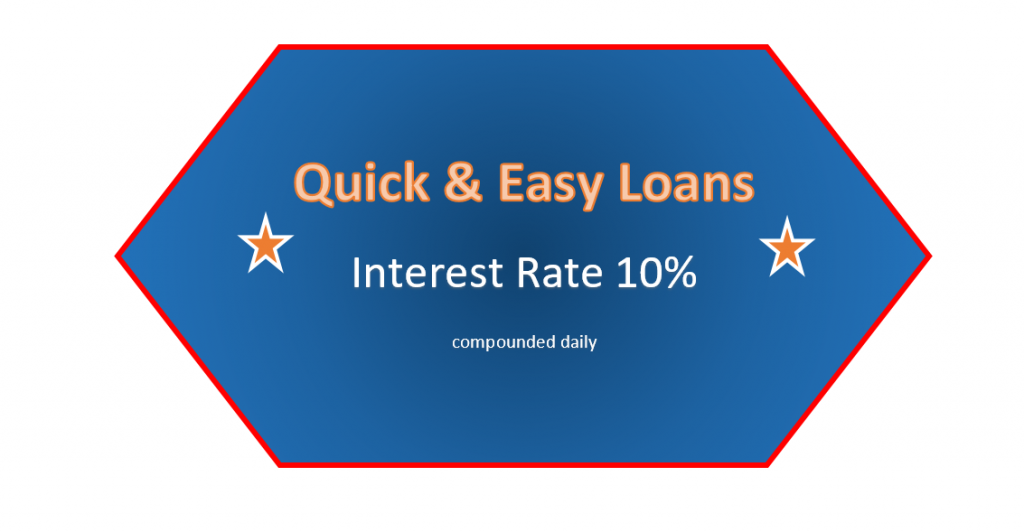Learning Objectives

By the end of this section it is expected that you will be able to:

• State the difference between nominal and effective interest rates
• Determine the effective annual interest rate
• Determine the best option when comparing nominal interest rates under different situations

# Nominal and Effective Rates of Interest

As consumers and investors we are inundated with all kinds of offers for both investments and financing. When we require a loan we seek the lowest possible interest rate but  when we invest we want the highest rate of return. As we search for the best offer it is important to recognize that the advertised interest rate is not necessarily the true interest rate.

An advertised rate of 10% with annual compounding works out to be equivalent to a rate of 10% annually. We refer to this stated rate of 10% as the nominal interest rate.  An advertised rate of 10% with daily compounding works out to be equivalent to a rate of 10.52%  since interest is calculated on the interest 365 times in one year. We refer to the 10.52% as the effective interest rate.

The stated interest rate is referred to as the nominal interest rate, as it describes the named or numerical value. It is the rate that is usually stated in advertisements. The actual interest rate, or effective interest rate, reflects the real rate of return as it takes the compounding periods into account.

With simple interest calculations (where there is no compounding),  the stated annual interest rate indicates the true rate of return. If $1000 is borrowed at 6% for one year, then the interest owined will be I = Prt =$1000 × 0.06/yr × 1yr = $60. With compound interest calculations, the stated annual interest rate does not indicate the true interest cost. If$1000 is invested at 6% for one year compounded semiannually, then the interest owed will be  $60.90 rather than$60.

One way to determine the  effective interest rate is to divide the total compound interest for the first year by the principal amount. If in the first year $60.90 is the interest charged on a principal of$1000 then the effective interest rate is  $60.90/$1000 = 0.0609 = 6.09%.  Although the nominal interest rate is 6%, the effective rate is 6.09%.

It is also possible to use a formula to calculate the effective interest rate.

Effective Interest Rate Formula

The effective interest rate formula is: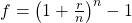where f = effective interest rate r = nominal interest rate (annual interest rate) n = number of times in one year that interest is calculated

EXAMPLE 1

Use the formula to determine the effective interest  rate for 6% compounded annually.

Solution

 f = ? r = 6% = 0.06 n = 1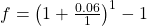Replace the variables with their values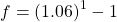Add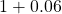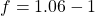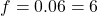The effective interest rate is 6%. Note that the nominal and effective rate are the same since the number of compounding period is one (n = 1).

TRY IT 1

What is the effective rate of 4% compounded yearly?

4%

EXAMPLE 2

Use the formula to determine the effective interest  rate for 6% compounded monthly.

Solution

 f = ? r = 6% = 0.06 n = 12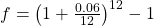Replace the variables with their values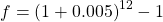Divide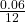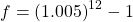Add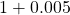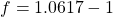Raise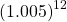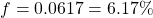The effective interest rate is 6.17%

TRY IT 2

What is the effective rate of 4% compounded monthly?

4.07%

EXAMPLE 3

What is the effective rate for a nominal rate of 9.8% compounded weekly?

Solution

 f = ? r = 9.8% = 0.098 n = 52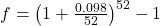Replace the variables with their values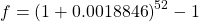Divide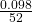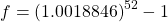Add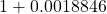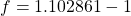Raise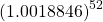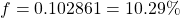The effective interest rate is 10.29%

TRY IT 3

Determine the effective rate of interest on a loan that is advertised at a rate of 7.8% compounded daily.

8.11%

It is important to consider the effective interest rate, rather than the nominal rate,  when deciding on investments or loans.

Consider Bank A which offers a savings plan at 6.25% compounded monthly and Bank B which offers 6.5% compounded semi-annually.  Which of the two banks offers the better rate of return?  Although both banks offer the same nominal interest rate, their effective rates differ.  The effective rate will reflect the actual rate of return in one year. Example 4 will illustrate this.

EXAMPLE 4

Bank A offers 6.25% compounded monthly while Bank B offers 6.5% compounded semi-annually.  Which bank offers the better effective rate of return?

Solution

For Bank A:

 f = ? r = 6.25% = 0.0625 n = 12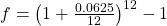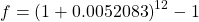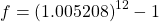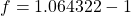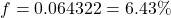For Bank B:

 f = ? r = 6.5% = 0.065 n = 2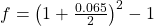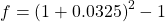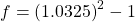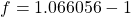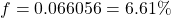Note that Bank A’s effective rate, 6.43%, is less than both Bank B’s nominal rate of 6.5% and Bank B’s effective rate of 6.61%. Bank B offers the better effective rate of return.

TRY IT 4

Sam plans to invest a lottery win of  $15,000. He is considering two different options. Option A offers 3.56% compounded weekly and Option B offers 3.48% componded monthly. Which option offers a better rate of return? Show answer Option A 3.62%; Option B 3.54%; Option A offers a better rate of return. EXAMPLE 5 Consider two options for a 2 year loan. Bank A will charge 7.2% compounded monthly while Bank B will charge 7.4% compounded semi-annually. Which bank offers the less expensive loan (charges the lower effective rate)? Solution For Bank A:  f = ? r = 7.2% = 0.072 n = 12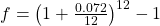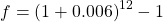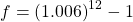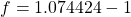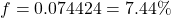For Bank B:  f = ? r = 7.4% = 0.074 n = 2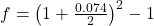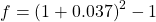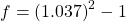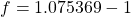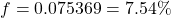Bank A’s effective rate, 7.44%, is less than Bank B’s effective rate of 7.54. By a slight margin, Bank A offers the less expensive loan. TRY IT 5 Sam needs to borrow$5500. He is offered two different loans. One loan is  at a bank for 6.8% compounded quarterly and the other is at a credit union for 6.9% compounded semiannually.

Which is the better option for Sam?

Bank 6.98%; Credit Union 7.02%; the Bank is a slightly better option for a loan.

# Key Concepts

• to determine the effective annual interest rate (f):
•• when investing money you want the higher effective interest rate; when borrowing money you want the lower effective interest rate.

# Glossary

effective interest rate

takes the compounding periods into effect so it is a better reflection of the actual interest charges.

nominal interest rate

is normally the stated rate. It does not take the compounding periods into effect.

# 9.3 Exercise Set

1. Determine the effective interest rates (rounded to two decimal places) for the following nominal interest rates when there is monthly compounding.
1. 8%
2. 3.7%
3. 2.64%
4. 5%
2. Determine the effective interest rates (rounded to two decimal places) for the following nominal interest rates when there is daily compounding.
1. 8%
2. 3.7%
3. 2.64%
4. 5%
3. Determine the effectiveinterest rate (rounded to two decimal places) when 10% is compounded
1. Yearly
2. Semi-annually
3. Quarterly
4. Monthly
5. Weekly
6. Daily
4. You have a choice between purchasing a savings certificate offering 3.6% simple interest or putting your money in a savings account at 3.6% compounded monthly. What is the difference between the effective  rates?
5. What simple interest rate would give you the same return as
1. 6% compounded daily?
2. 5% componded semiannually?
3. 4.2% componded weekly?
1. What is the effective interest rate?
2. What total amount do you owe in four years?
3. What amount of this will be the interest charged?You borrow $4800 to be paid back in 4 years at a rate of 4.4% componded quarterly. 6. You are needing to borrow$10,000 to be paid back over a 3 year period and you are consider two options. With Option A the interest rate is 3.5% compounded daily and with Option B the interest rate is 3.52% componded semiannually.  Which option offers the less expensive loan (charges the lower effective rate)?
7. You invest $5600 for two years at a rate of 5.2% componded monthly. 1. What is the effective interest rate? 2. What total amount will be in your account after two years? 3. What amount of this will be the interest earned? 8. You can invest$2000 for one year under the following two options:  Option A  6.2 % simple interest or Option B  6.15% compounded weekly.  For each of these
1. Determine the effective interest rate.
2. Determine the compound amount at the end of one year.
3. Determine the interest that is earned.
9. L. Shark offers to lend you $1000 for one year at 50% interest compounded daily. 1. What is the effective rate of interest on this loan (rounded to the nearest hundred 2. What total amount do you owe at the end of one year? 3. What is the interest component? 4. What would the interest component be if instead you were charged 50% simple interest? # Answers 1. 8.30% 2. 3.76% 3. 2.67% 4. 5.12% 1. 8.33% 2. 3.77% 3. 2.68% 4. 5.13% 1. 10% 2. 10.25% 3. 10.38% 4. 10.47% 5. 10.51% 6. 10.52% 1. The effective rate for 3.6% simple interest is 3.6%. The effective rate for 3.6% compounded monthly is 3.66% so a difference of 0.06%. 1. 6.18% 2. 5.06% 3. 4.28% 1. 4.47% 2.$5718.21
3. $918.21 2. Option A the effective rate is 3.56%; Option B the effective rate is 3.55%. Option B is less expensive by 0.01% 1. 5.33% 2.$6212.37
3. $612.37 1. Option A 6.2% and Option B 6.34% 2. Option A$2124 and Option B  $2126.78 3. Option A$124 and Option B  $126.78 1. 64.82% 2.$1648.16
3. $648.16 4.$500